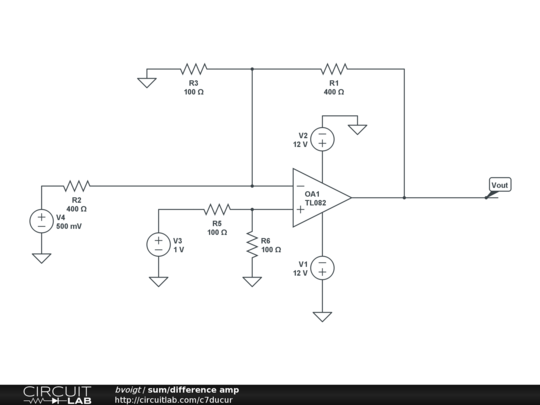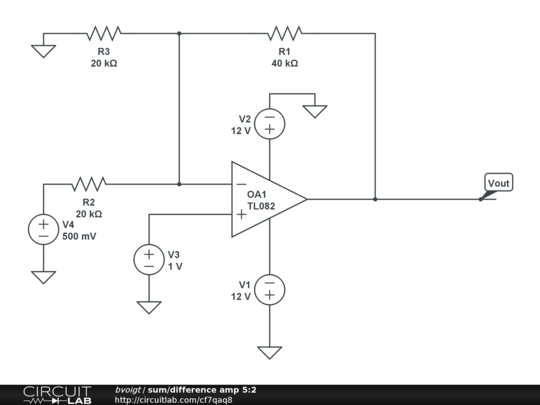# differencing op amp circuit

How would I implement an differencing op amp circuit that performs the operation 5*v1-2*v2 with the op amp power supply being 12vdc? I already tried the basic circuit but I got negative resistance. Is that normal?

Schematic:• Why don't you post the schematic of what you tried? I have no idea what you mean when you say you got negative resistance. – Matt Young Mar 5 '13 at 19:35
• @Matt: When he calculated R3, he apparently got a negative value. – Ben Voigt Mar 5 '13 at 19:58
• So I see... So what he's trying to get is a difference amplifier that runs off a 12V single supply. What is shown here will not come anywhere close to that. Answer enroute... – Matt Young Mar 5 '13 at 20:00
• I still got a negative value for R3 for some reason – user1047968 Mar 5 '13 at 20:01
• Is this single supply, or split? – Matt Young Mar 5 '13 at 20:12

What you have now is an analog comparator due to positive feedback. Diagram your circuit again, this time using negative feedback, and when you repeat the calculations you'll get much more reasonable resistor values.

(If you're copying from a textbook sum/difference amp circuit, please note that having the feedback resistor top or bottom is not important, connecting it to the inverting input pin is, and that op-amp symbols vary in placement of the input pins. Your textbook likely drew the circuit with pin 2 above pin 3.)

Of course, with a 12V supply, your inputs will have to be chosen so that the output isn't 40V.

You might try a topology like this (resistor values are not set correctly, that's up to you):(And for Matt's benefit, here's the solution, showing that R3 is not unnecessarily adding complication)A nice way to analyze this is to first consider the circuit with only $R1$ and $R2$ present. Then

$$V_{out} = (1 + \frac{R1}{R2}) V3 - \frac{R1}{R2} V4$$

If the non-inverting gain is too high, use a voltage divider on $V3$. (This gives the Wikipedia circuit).

If the inverting gain is too high, use a voltage divider on $V4$.

Final gains:

$$G = \frac{R1}{R2 || R3} = \frac{R1 R2 R3}{R2 + R3}$$ $$V_{out} = (G+1) \frac{R5}{R5 + R6} V3 - G \frac{R3}{R2 + R3} V4$$

• I'm new at this but I used the text book formula to find the resistance values, when I solved for R3, I got the negative value. – user1047968 Mar 5 '13 at 20:04
• @user1047968: First, fix your schematic. Then, apply the ideal op-amp rules (input current is zero, input voltages are equal) and solve Kirchoff's Current and Voltage Laws. You get to pick one or two of the resistor values, for example, R4 as 1k. – Ben Voigt Mar 5 '13 at 20:05
• It's not that simple with a single supply. – Matt Young Mar 5 '13 at 20:08
• @Matt: Neither the question nor the circuit indicate single supply. – Ben Voigt Mar 5 '13 at 20:09
• The question states "with the op amp power supply being 12vdc," he needs to clarify this point. The schematic indicates nothing useful to me other than a fundamental lack of understanding. – Matt Young Mar 5 '13 at 20:12